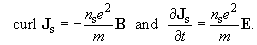Science, Maths & Technology

### Become an OU studentSuperconductivity

Start this free course now. Just create an account and sign in. Enrol and complete the course for a free statement of participation or digital badge if available.

# Conclusion

Section 1 Superconductivity was discovered in 1911, and in the century since then there have been many developments in knowledge of the properties of superconductors and the materials that become superconducting, in the theoretical understanding of superconductivity, and in the applications of superconductors.

Section 2 A superconductor has zero resistance to flow of electric current, and can sustain a current indefinitely. The magnetic flux remains constant in a completely superconducting circuit, since changes in the flux from the field applied to the circuit are balanced by changes to (persistent) currents induced in the circuit. For each superconductor there is a critical temperature T﻿c below which the material is superconducting.

Superconductors also exhibit perfect diamagnetism, with B = 0 in the bulk of the material. The exclusion of magnetic field is known as the Meissner effect. An external magnetic field penetrates for a short distance into the surface of a superconducting material, and a current flows in the surface layer to screen the interior of the material from the applied field. Superconductivity is destroyed when the magnetic field strength exceeds a critical value for the material. The critical field strength falls to zero as the temperature is raised to the critical temperature. A superconducting specimen will have a critical current I﻿c above which the material reverts to the normal state. This critical current corresponds to the field strength exceeding the critical field strength in some region of the specimen.

Section 3 The two-fluid model of a superconductor regards some of the conduction electrons as behaving like normal electrons and some like superconducting electrons. For T ≪ T﻿c, all of the conduction electrons are superconducting electrons, but the proportion of superconducting electrons drops to zero at the critical temperature.

For a perfect conductor (which has R = 0), Maxwell's equations predict that the magnetic field cannot change, except in a thin surface layer. This does not predict the Meissner effect. The London equations are relationships between current density and magnetic field that are consistent with the Meissner effect:When combined with Maxwell's equations, they lead to the prediction that the magnetic field strength and the surface current decrease exponentially below the surface of a superconductor, over a characteristic distance called the penetration depth λ, which is typically tens of nanometres. The London equations are local relationships and therefore are strictly valid only when λ ≫ ξ, where the coherence length ξ is the characteristic distance over which n﻿s varies.

Section 4 There are two types of superconductors, type-I and type-II. For a type-I material in the form of a thin specimen parallel to the field, there is an abrupt transition to the normal state at the critical field strength B﻿c. When the field is inclined to the surface of a type-I material, the material exists in the intermediate state over a range of field strengths below B﻿c. In this state there are thin layers of normal and superconducting material, with the proportion of normal material rising to unity at field strength B﻿c. In type-I materials, the coherence length ξ is greater than the penetration depth λ, and the surface energy of the boundary between superconducting and normal material is positive, which favours a course subdivision into regions of normal and superconducting material.

A type-II superconductor has two critical field strengths, B﻿c1 and B﻿c2, between which the material is in the mixed state. In this state the superconductor is threaded by thin cores of normal material, through which the magnetic field passes. The coherence length ξ is shorter than the penetration depth λ, and the surface energy of the boundary between superconducting and normal material is negative, which favours a fine subdivision into regions of normal and superconducting material. To take advantage of the high values of Bc2 to produce high magnetic fields with superconducting magnets, it is essential to pin the normal cores to inhibit their motion.